# ISEE Upper Level Quantitative : Triangles

## Example Questions

### Example Question #1 : How To Find The Area Of An Equilateral Triangle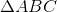is an equilateral triangle. Points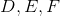are the midpoints of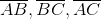, respectively.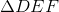is constructed.

Which is the greater quantity?

(a) The area of(b) Twice the area of(a) is greater.

(b) is greater.

It is impossible to tell from the information given.

(a) and (b) are equal.

(a) is greater.

Explanation:

If segments are constructed in which the endpoints form the midpoints of the sides of a triangle, then four triangles, congruent to each other and similar to the larger triangle, are formed. Therefore, one of these triangles - specifically,- would have one-fourth the area of. This meanshas more than twice the area of.

Note that the fact that the triangle is equilateral is irrelevant.

### Example Question #1 : How To Find The Length Of The Side Of An Equilateral Triangle

Which of the following could be the three sidelengths of an equilateral triangle?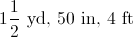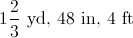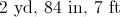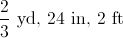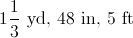Explanation:

By definition, an equilateral triangle has three sides of equal length. We can identify the equilateral triangle by converting the given sidelengths to the same units and comparing them.

We can eliminate the following by showing that at least two sidelengths differ.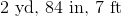2 yards =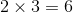feet.

Two sides have lengths 6 feet and 7 feet, so we can eliminate this choice.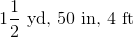4 feet =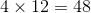inches

Two sides have lengths 48 inches and 50 inches, so we can eliminate this choice.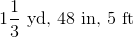5 feet =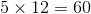inches

Two sides have lengths 48 inches and 60 inches, so we can eliminate this choice.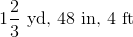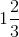yards =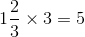feet

Two sides have lengths 4 feet and 5 feet, so we can eliminate this choice.yards =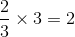feet =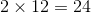inches

All three sides have the same length, making this the triangle equilateral. This choice is correct.

### Example Question #1 : Right Triangles

What is the hypotenuse of a right triangle with sides 5 and 8?

12

√89

15

5√4

√89

Explanation:

Because this is a right triangle, we can use the Pythagorean Theorem which says a2 + b2 = c2, or the squares of the two sides of a right triangle must equal the square of the hypotenuse. Here we have a = 5 and b = 8.

a2 + b2 = c2

52 + 82 = c2

25 + 64 = c2

89 = c2

c = √89

### Example Question #2 : How To Find The Length Of The Hypotenuse Of A Right Triangle : Pythagorean Theorem

Which is the greater quantity?

(a) The hypotenuse of a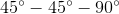right triangle with a leg of length 20

(b) The hypotenuse of a right triangle with legs of length 19 and 21

(a) and (b) are equal

It is impossible to tell from the information given

(b) is greater

(a) is greater

(b) is greater

Explanation:

The hypotenuses of the triangles measure as follows:

(a)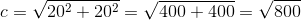(b)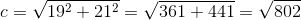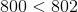, so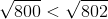, making (b) the greater quantity

### Example Question #1 : How To Find The Length Of The Hypotenuse Of A Right Triangle : Pythagorean Theorem

Which is the greater quantity?

(a) The hypotenuse of a right triangle with legsand.

(b) The hypotenuse of a right triangle with legsand.

It is impossible to tell from the information given.

(b) is greater.

(a) is greater.

(a) and (b) are equal.

(a) is greater.

Explanation:

The hypotenuses of the triangles measure as follows:

(a)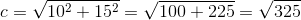(b)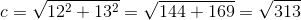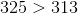, so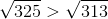, making (a) the greater quantity.

### Example Question #1 : How To Find The Length Of The Hypotenuse Of A Right Triangle : Pythagorean Theorem

A right triangle has a leg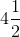feet long and a hypotenuse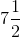feet long. Which is the greater quantity?

(a) The length of the second leg of the triangle

(b) 60 inches

It is impossible to tell from the information given.

(b) is greater.

(a) and (b) are equal.

(a) is greater.

(a) is greater.

Explanation:

The length of the second leg can be calculated using the Pythagorean Theorem. Set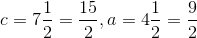: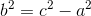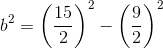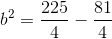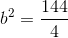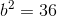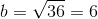The second leg therefore measures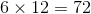inches.

### Example Question #1 : How To Find The Length Of The Hypotenuse Of A Right Triangle : Pythagorean Theorem

What is the hypotenuse of a right triangle with sides 9 inches and 12 inches?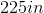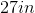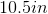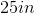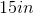Explanation:

Since we're dealing with right triangles, we can use the Pythagorean Theorem (). In this formula, a and b are the sides, while c is the hypotenuse. The hypotenuse of a right triangle is the longest side and the side that is opposite the right angle. Now, we can plug into our formula, which looks like this: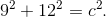We simplify and get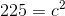. At this point, isolate c. This means taking the square root of both sides so that your answer is 15in.

### Example Question #1 : How To Find The Length Of The Hypotenuse Of A Right Triangle : Pythagorean Theorem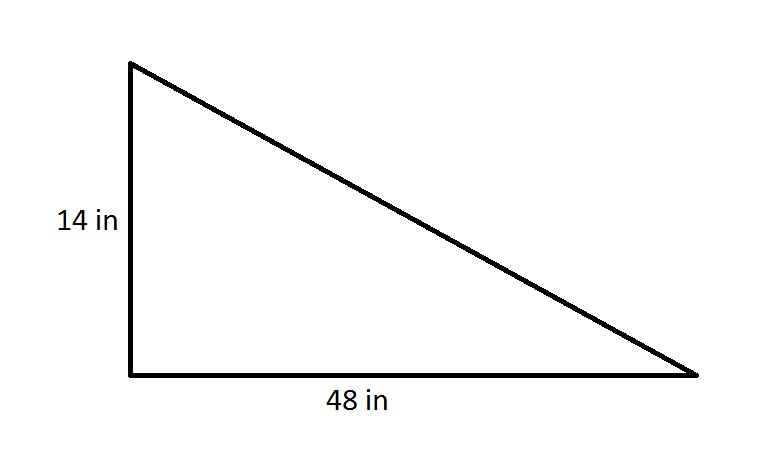The perimeter of a regular pentagon is 75% of that of the triangle in the above diagram. Which is the greater quantity?

(A) The length of one side of the pentagon

(B) One and one-half feet

(A) and (B) are equal

It is impossible to determine which is greater from the information given

(B) is greater

(A) is greater

(B) is greater

Explanation:

By the Pythagorean Theorem, the hypotenuse of the right triangle is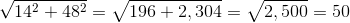inches, making its perimeter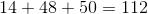inches.

The pentagon in question has sides of length 75% of 112, or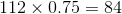.

Since a pentagon has five sides of equal length, each side will have measure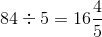inches.

One and a half feet are equivalent to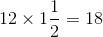inches, so (B) is the greater quantity.

### Example Question #32 : Isee Upper Level (Grades 9 12) Quantitative Reasoning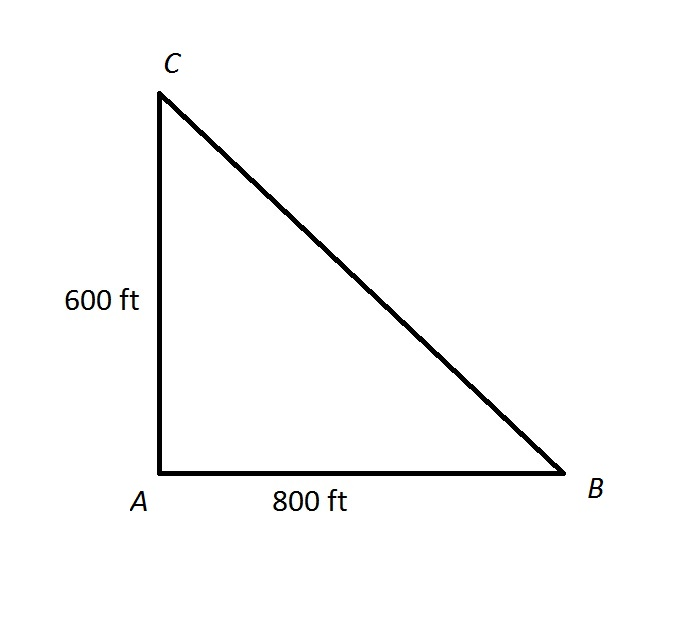The track at Gauss High School is unusual in that it is shaped like a right triangle, as shown above.

Cary decides to get some exercise by running from point A to point B, then running half of the distance from point B to point C.

Which is the greater quantity?

(A) The distance Cary runs

(B) One-fourth of a mile

It is impossible to determine which is greater from the information given

(B) is greater

(A) and (B) are equal

(A) is greater

(B) is greater

Explanation:

By the Pythagorean Theorem, the distance from B to C is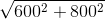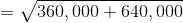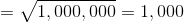feet

Cary runs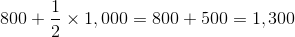feet

Since 5,280 feet make a mile, one-fourth of a mile is equal to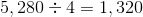feet.

(B) is greater

### Example Question #8 : How To Find The Length Of The Hypotenuse Of A Right Triangle : Pythagorean Theorem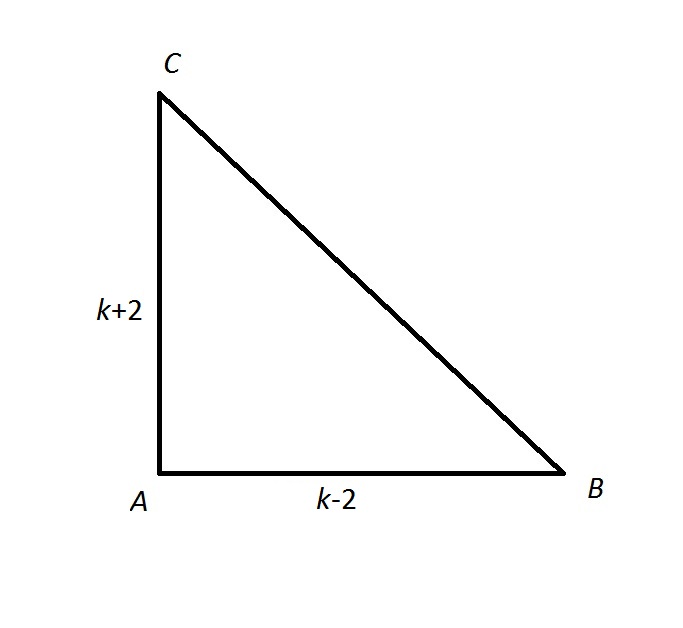Give the length of the hypotenuse of the above right triangle in terms of.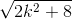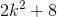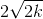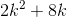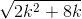Explanation:

If we letbe the length of the hypotenuse, then by the Pythagorean theorem,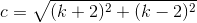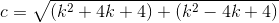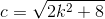### All ISEE Upper Level Quantitative Resources